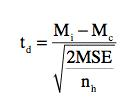# Dunnetts Test

Posted in Statistics, Total Reads: 2740

## Definition: Dunnetts Test

The statistical procedure for comparing each experimental mean with the control mean is called "Dunnett's test". It is so named after the statistician who developed it.

Dunnett's test controls the Experimental Error Rate (ERR) (When a series of significance tests is conducted, the Experiment wise Error Rate (EER) is the probability that one or more of the significance tests results in a Type 1 error) and is more effective than tests designed to compare each mean with each other mean. Dunnett's test is conducted by computing a t-test between each experimental group and the control group using the formula:where Mi is the mean of the ith experimental group, Mc is the mean of the control group, MSE is the mean square error as calculated from the ANOVA tableand nh is the harmonic of the sample sizes of the experimental group and the control group.

Hence, this concludes the definition of Dunnetts Test along with its overview.

Browse the definition and meaning of more terms similar to Dunnetts Test. The Management Dictionary covers over 7000 business concepts from 6 categories.

Search & Explore : Management Dictionary

Similar Definitions from same Category: